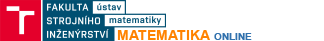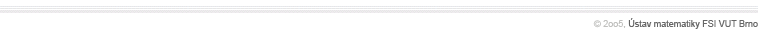Convergence of seriesApplication of the ratio, root or integral test on a series.Calculation of the Taylor seriesCalculates and draw the graph of the first n elements of the Taylor series for an entered function.Calculation of the Fourier seriesCalculates and draw the graph of the first n elements of the Fourier series for an entered function.Direction fieldDraws the direction field for a differential equation $y'=f(x,y)$.Direction field for a system of equationsDraws the direction field for a system of two differential equations $y_1'=f(y_1,y_2)$$y_2'=g(y_1,y_2)$Solution in the direction fieldDraws the  direction field and several different solutions for a differential equation $y'=f(x,y)$.Separation of variablesGeneral solution of a differential equation which can be written in the form $y'=f(x)g(y)$.Linear differential equation of the first orderGeneral solution of a differential equation which can be written in the form $y'+py=q$.Homogeneous linear ODEs with constant coefficientsCalculation of the basis of the space of solutions of a homogeneous linear ODE with constant coefficients.Finite difference method for the Poisson equationBoundary value problem.$-\frac{\partial^2 u}{\partial x^2}-\frac{\partial^2 u}{\partial y^2}=f(x,y)$ inside the square $\Omega = [0,1]x[0,1]$, $u=0$ on the boundary $\partial \Omega = \Gamma$,is being solved by the finite difference method. $n$ inner nodes on the side of the square generates a system of $n^2$ linear equations.